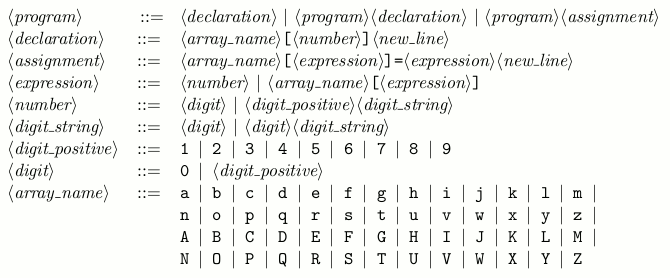Time Limit : sec, Memory Limit : KB
English

# Problem H: Bug Hunt

In this problem, we consider a simple programming language that has only declarations of one- dimensional integer arrays and assignment statements. The problem is to find a bug in the given program.

The syntax of this language is given in BNF as follows:where <new line> denotes a new line character (LF).

Characters used in a program are alphabetical letters, decimal digits, =, [, ] and new line characters. No other characters appear in a program.

A declaration declares an array and specifies its length. Valid indices of an array of length n are integers between 0 and n - 1, inclusive. Note that the array names are case sensitive, i.e. array a and array A are different arrays. The initial value of each element in the declared array is undefined.

For example, array a of length 10 and array b of length 5 are declared respectively as follows.

a
b


An expression evaluates to a non-negative integer. A <number> is interpreted as a decimal integer. An <array_name> [<expression>] evaluates to the value of the <expression> -th element of the array. An assignment assigns the value denoted by the right hand side to the array element specified by the left hand side.

Examples of assignments are as follows.

a=3
a=0
a=a[a]
a[a]=a


A program is executed from the first line, line by line. You can assume that an array is declared once and only once before any of its element is assigned or referred to.

Given a program, you are requested to find the following bugs.

• An index of an array is invalid.
• An array element that has not been assigned before is referred to in an assignment as an index of array or as the value to be assigned.

You can assume that other bugs, such as syntax errors, do not appear. You can also assume that integers represented by <number>s are between 0 and 231 - 1 (= 2147483647), inclusive.

## Input

The input consists of multiple datasets followed by a line which contains only a single '.' (period). Each dataset consists of a program also followed by a line which contains only a single '.' (period). A program does not exceed 1000 lines. Any line does not exceed 80 characters excluding a new line character.

## Output

For each program in the input, you should answer the line number of the assignment in which the first bug appears. The line numbers start with 1 for each program. If the program does not have a bug, you should answer zero. The output should not contain extra characters such as spaces.

## Sample Input

a
a=a
.
x
x=x
.
a
a=1
.
b
b=2
b=b[b]
b=b
.
g
G
g=0
g=G
.
a
a=1
B
B[a]=2
a[B[a]]=3
a=a
.
.


## Output for the Sample Input

2
2
2
3
4
0